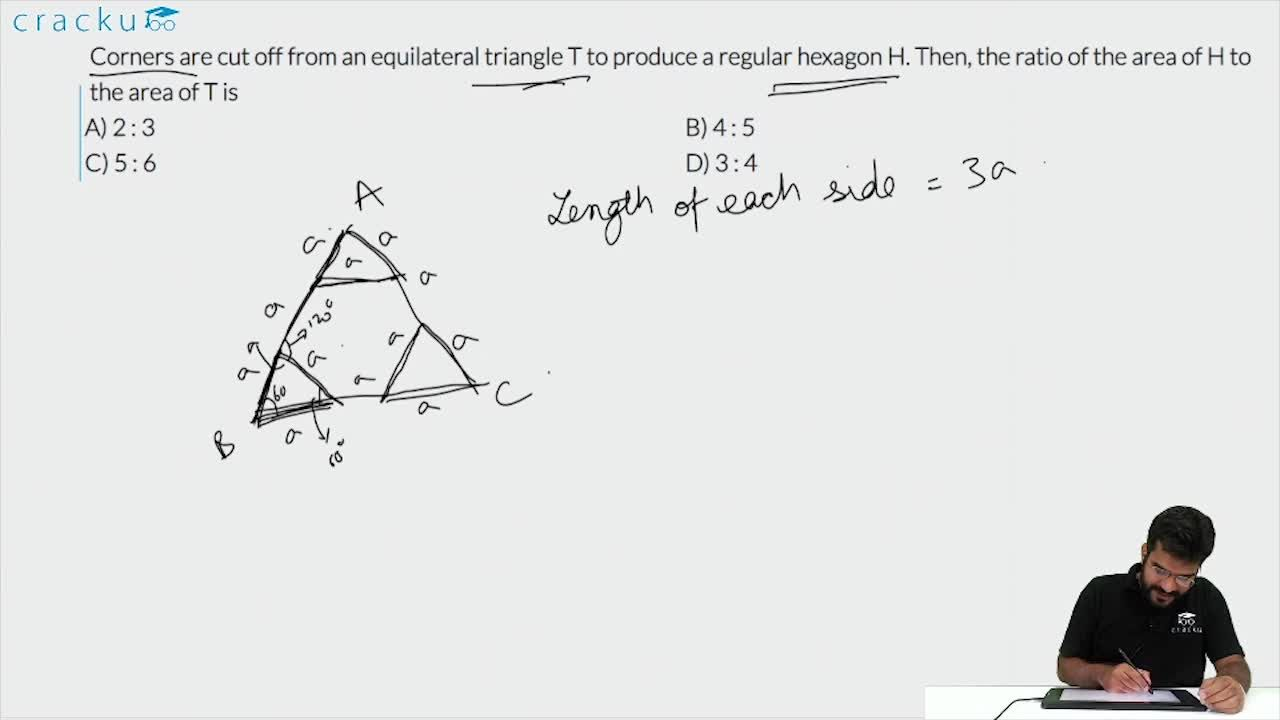Question 28

# Corners are cut off from an equilateral triangle T to produce a regular hexagon H. Then, the ratio of the area of H to the area of T is

Solution

The given figure can be divided into 9 regions or equilateral triangles of equal areas as shown below,

Now the hexagon consists of 6 regions and the triangle consists of 9 regions.

Hence the ratio of areas = 6/9 =2:3

### View Video Solution